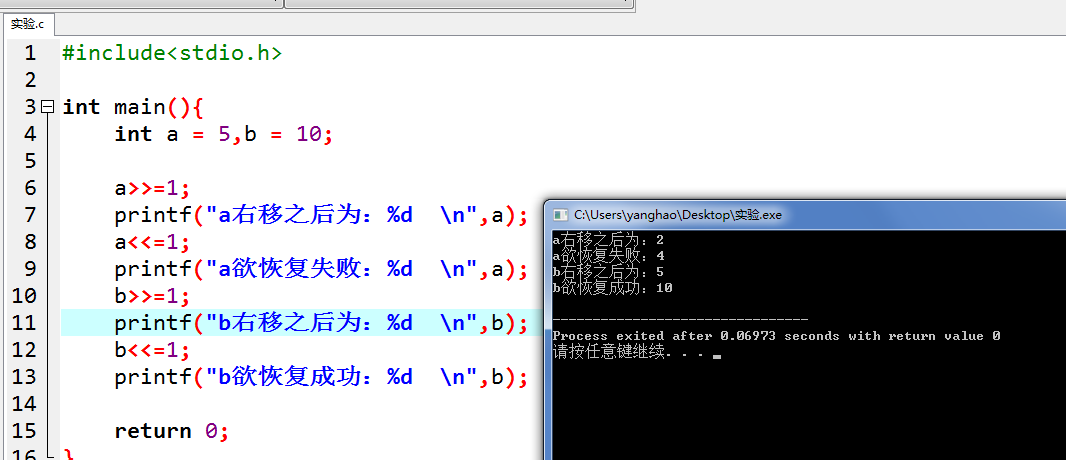• 下来分享一下写的一个关于交换两个数C语言代码。可能这些还存在很大的问题，希望大家可以指正。方法一：允许创建第三个变量#include int main() {  int a=10;  int b=20;  int tmp=a;  a=b;  b=tmp;  ...

今天是第一次接触C语言，之前有一点C++的基础，所以学习起来还挺顺手的。下来分享一下写的一个关于交换两个数的C语言代码。可能这些还存在很大的问题，希望大家可以指正。
方法一：允许创建第三个变量
#include<stdio.h>
int main()
{
int a=10;
int b=20;
int tmp=a;
a=b;
b=tmp;
printf("%d\n",a);
printf("%d\n",b);
return 0;
}
方法二：不创建新的变量，利用两个变量之间的关系，例如异或，加减乘除，但考虑到加减乘除预算可能会出现溢出情况，故采用异或是最保险的。
#include<stdio.h>
int main()
{
int a=3;
int b=5;
a=a^b;
b=a^b;
a=a^b;
printf("%d\n",a);
printf("%d\n",b);
return 0;
}
但综合这两种方法，方法一中创建了一个临时变量，这样的做法让程序简单易读，并且执行起来速度也快一点；方法二虽然看起来很完美 ，但实际操作起来并不推崇这种写法，会加大程序运行时间。

本文出自 “Stand out or Get out” 博客，请务必保留此出处http://jiazhenzhen.blog.51cto.com/10781724/1702318
展开全文• //指针变量交换两个数，函数内部要交换两个数要通过指针交换 { int t = *x; *x = *y; *y = t; } 2.异或法 根据将某个与另一个连续与或两次，可得到本身。将两个进行与或运算，再分别与...
1. 临时变量法
创建一个临时变量先保存一个数的值，然后再进行交换赋值，最后将临时变量的值赋给另一个变量
void swap(int *x, int *y)
//指针变量交换两个数的值，函数内部要交换两个数的值要通过指针交换
{
int t = *x;
*x = *y;
*y = t;
}

2.异或法
根据将某个数与另一个数连续与或两次，可得到本身。将两个数进行与或运算，再分别与本身做与或运算便可得到另一个数
void swap1(int *x, int *y)
//不创建临时变量,交换两个数的值(异或法)：一个数于任何一个数与或两次，得到的数等于本身
{
*x = *x^*y;
*y = *x^*y;//(*x^*y)^*y=*x(原值)
*x = *x^*y;//(*x^*y)^((*x^*y)^*y)=*y（原值）
}

注：该方法相比其他方法不用创建临时变量，切不会出现越界
3.加减法
两个数相加再减去另一个数可得到原来的数；
void swap2(int *x, int *y)
{
*x = *x + *y;//此处有可能越界
*y = *x - *y;
*x = *x - *y;
}

注：*x+y的大小不能确定，有可能超出类型范围；因此该方法正确运行的前提是x+*y不能超出类型范围，类似的方法还有乘除法，不过该方法比加减法更容易越界，因此不再过多介绍。下面给出代码：
void swap(int *p1, int *p2)
{
*p1 = *p1*(*p2);
*p2 = *p1 / (*p2);
*p1 = *p1 / (*p2);
}


展开全文swap函数 异或法
• C语言交换两个数的方法 方法一：利用中间变量 int a =10,b=20; int tmp; tmp = a; a = b; b = tmp; 方法二：利用迭代 int a = 10,b=20; a = a+b; b = a-b; a = a-b;
C语言中交换两个数的方法  方法一：利用中间变量
int  a =10,b=20;
int tmp;
tmp = a;
a = b;
b = tmp;
方法二：利用迭代
int a = 10,b=20;
a = a+b;
b = a-b;
a = a-b;
展开全文• 对于C语言交换两个数的方法，目前大体上有这么5种：1：直接利用中间变量进行交换；2：利用指针传入函数地址在函数内部进行利用中间变量交换；3：将两个需要交换的进行加减运算进行交换；注：因C语言有浮点数之分...对于C语言中交换两个数的方法，目前大体上有这么5种：
1：直接利用中间变量进行交换；
2：利用指针传入函数地址在函数内部进行利用中间变量交换；
3：将两个需要交换的数进行加减运算进行交换；
注：因C语言有浮点数之分，不可以认为乘除也可进行交换。
4：将两个数进行亦或运算，也可以实现交换两个数的目的；
5：对两个数进行位操作，也可以实现交换两个数的目的。
接下来我将对后面三种进行分析：
前面两种太过常见，在此不做说明：
第三种：加减交换
将两个数之和赋值给第一个数，然后用新第一个数减去第二个数得到旧第一个数，并赋值给第二个数
，再用新第一个数减去新第二个数(旧第一个数)得到旧第二个数，并赋值给第一个数，在此，实现
两个数的交换，代码如下：
1 #include
2
3 intmain(){
4 int a=10,b=20;
5 a+=b;
6 b=a-b;
7 a=a-b;
8
9 return 0;
10 }
注意：此法使用缺陷
当需要交换的两个数是指向同一下标的数组元素，那么此时，此法失效。
诸君请看：
1 #include
2
3 intmain(){4 int a = {1,2,3,4,5};5 int i=2,j=2;6 a[i] += a[j]; //因为i=j;所以此时a[i]=a[j]=2[i];
7 a[j] = a[i] - a[j];//由上知，此时a[i]=a[j]=0;
8 a[i] = a[i] - a[j];//此时两者均为0；交换失败9　　　return 0;
10 }
此外，使用此法时应注意两个数之和不能超过所定义的类型所能表达的最大数，不然也将交换失败
第四种：异或运算进行交换
在此，我先说一下异或运算的原理：两个数做异或运算，将两个数化为二进制，逐位比较，相同者得0，不同者得1；具体如下：
0 1 0 1   1 0 0 1
1 0 0 1   0 1 0 0
运算得　　　1 1 0 0   1 1 0 1
0 1 0 1   1 0 0 1
与第一个数   　　　　　　　　　　　　　　至此感悟，代码如下：
运算得       1 0 0 1   0 1 0 0
1 #include
2
3 intmain(){4 int a = 10,b = 20;5 b ^=a;6 a ^=b;7 b ^=a;8
9 return 0;10 }
注：和上一方法一样，此法在对数组使用时也受到相同的限制，但只需在对数组使用之前做一个，判断确保指向的不是同一个数，
那么我觉得这种方法应是最简单快捷的。
第五种：位运算进行交换
该方法也是基于二进制进行运算
首先带大家认识两个位运算符：>> 箭头朝右指，故右移运算符   <
比如 5>>1 表示将5右移一位  -->  即    5的二进制 0 0 0 0  0 1 0 1    右移一位得   0 0 0 0   0 0 1 0  原本末尾的1被移走，高位补0；左移同理
注意：因右边是数据的最低位，所以当右移出去后，再左移是不能恢复到原来的数字的，但左移不同，左边剩下的高位都为0，都是存在的，当你的数
字不足够大时，左移之后是还可以通过右移恢复原数的当想使用位运算交换两个数的时，应先对每个数实际二进制位数做简单估测，再确定所移动位数，下面为交换两个数的实例
1 #include
2
3 intmain(){4 int a = 5,b = 10;5 a <<= 8; //将a移动到高位
6 a += b; //将b的值加到a移动之后的低位
7 b = a >> 8; //取a原来的值
8 a = a & 0xff ; //取新移进来的b，高位直接通过与运算赋值为0
9
10 return 0;11 }
注：上述代码中的&运算符运算原理和亦或相似，这个是如果两个都为1，则为1，否则为0；
当用此方法进行交换两个数时，一是两个 数的大小 比第三种方法还受限制，而且所移的位数不好确定，不推荐使用
第一次写博客，我知道肯定毛病颇多，望各位大佬口下留情，手下留情，多多指点；谢过谢过
标签：
版权申明：本站文章部分自网络，如有侵权，请联系：west999com@outlook.com
特别注意：本站所有转载文章言论不代表本站观点，本站所提供的摄影照片，插画，设计作品，如需使用，请与原作者联系，版权归原作者所有

展开全文• //需要传指针才能改变实参 void swap1(int* x, int* y) { int temp; temp = *x; *x = *y; ...void swap2(int* x, int* y) { //两个相同的异或为0，任何异或0还是它本身 *x = *x ^ *y; ...CSDN 新手
•   在开始学习C语言的过程中，我们一定都做过这样一道题，交换两个数。可能大部分人都会这道题，但今天我想试试用不同的方法做这道题。   1、创建临时变量 #include&lt;stdio.h&gt; int main() { ...
• 交换两个数 方法一：(创建临时变量来交换两个数） tem=a; a=b; b=tem; 方法二：（不创建临时变量） a=a+b; a 10~30 b 20 b=a-b; a 30 b 20~10 a=a-b; a 30~20 b 10 缺点：a+b有可能溢出 ...
• 在日常编程里面经常会遇到交换两个变量的内容的任务，对于泛型类型而言有两种泛型策略来实现，下面跟着小编一起来学习学习。
• c语言程序设计题目二：交换两个数 #include <stdio.h> #include <stdlib.h> int main() { double firstNumber, secondNumber, temporaryVariable; printf("输入第一个数字："); scanf("%lf", &...
• #include void swap(int *p ,int *p1){ int *temp ; temp = p; p= p1; p1 = temp; } void swap2(int *p ,int *p1){ int temp ; temp = *p; *p= *p1; *p1 = temp; } void main(){ ... int b =
• 三种方法交换两个数： 方法一：利用中间变量，以此依托交换两个数 方法二：利用两之间互相加减，来实现两的交换 方法三：利用按位异或^来实现两的交换，注意两需转换成二进制比较 #define _...
• 交换两个变量a、b的 代码如下： #include<stdio.h> int main() { void swap1(int p1,int p2); void swap2(int *p1,int *p2); int *p1,*p2,a,b; p1=&a; p2=&b; printf("请输入a、b的："); ...指针
• 以下两个数交换方式是以宏的方式呈现，写了两种方式 #include #define SWAP(a,b) {a=a+b;b=a-b;a=a-b;} int main(void) { int a=2,b=3; SWAP(a,b); printf("a=%d,b=%d\n",a,b); return 0; } #include ...
• 主要介绍了交换两个文本内容的C语言代码，有需要的朋友可以参考一下
• #define _CRT_SECURE_NO_WARNINGS #include void Swap(int *px,int *py) { int tmp = *px; *px = *py; *py = tmp; } int main() { int a = 1; int b = 2; Swap(&a,&b); printf("a = %
• C语言：给定两个整形变量的，将两个值的内容进行交换https://blog.csdn.net/lyf13132/article/details/84764950 用函数实现两个数交换，其本质上与基本的创建临时变量一样，只是把基本语句放在了函数里，然鹅要...
• ## C语言中交换两个数的方法

万次阅读 多人点赞 2017-05-06 11:35:07
问题描述：程序中有两个a,b,其中a=4,b=5，现在希望交换两个数，使得a=5,b=4.在这里我总结了一下目前我已经掌握的C语言交换两个数的方法，主要如下几种，下面我将分别来进行介绍。方法1： 使用一个临时变量来...
• C语言交换两个变量的可以在主函数中直接实现，也可以通过子函数来实现。下面我们介绍用子函数实现的情况。 1.将两个变量的作为实参直接传递，在子函数内部定义一个普通变量来对两个形参进行交换，并分别在子...指针
• 1.互换两个数字： # include&lt;stdio.h&gt; void g(int * p,int * q) { int t; //如果要互换*p和*q的,则t必须定义为int, t = * p; //不能定义为int*,否则会有语法错误. * p = * q; * q = t; } int ...
• 在这里我们既然不需要使用中间变量，那么我们就要对这里面进行一系列的思考，我们可以通过两个的和和差进行判断。 例：比如我们给出两个...代码如下：//不创建临时变量，交换两个数 #include #include<stdlib
• 指针交换两个数 突然看到自己以前研究关于指针交换两个数的代码，当时自己还通过不断的printf来观察地址的变化，理解了之后发现不论几级指针，其核心是不变的。 一级指针交换 void swap(int *p, int *q) { int...指针...

# 交换两个数的值c语言c语言 订阅